# Calculate Areas: Parallelograms

In this worksheet, students must calculate the areas of the given parallelograms.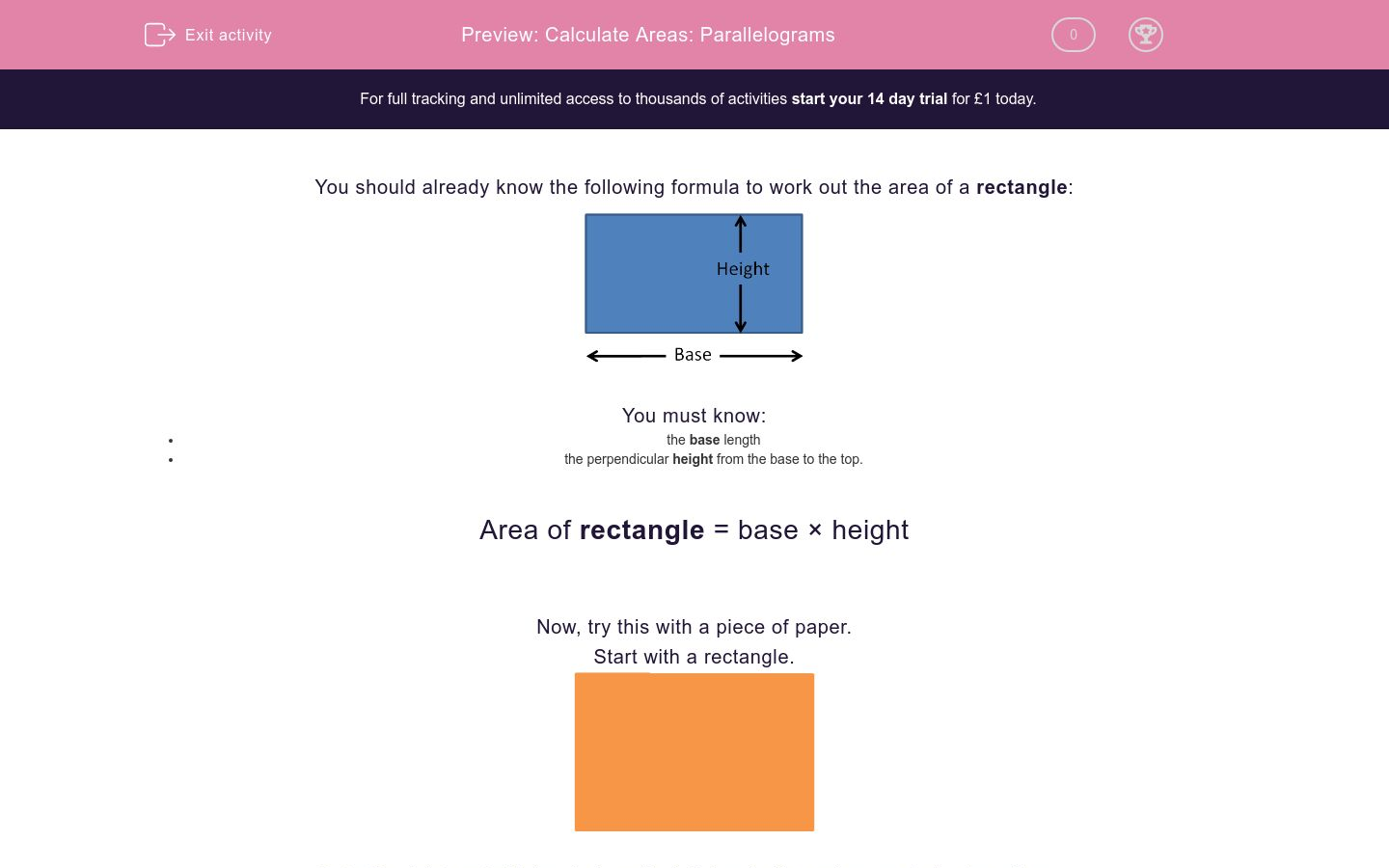Key stage:  KS 2

Curriculum topic:   Maths and Numerical Reasoning

Curriculum subtopic:   2D Shapes: Triangles, Quadrilaterals and Polygons

Difficulty level:### QUESTION 1 of 10

You should already know the following formula to work out the area of a rectangle: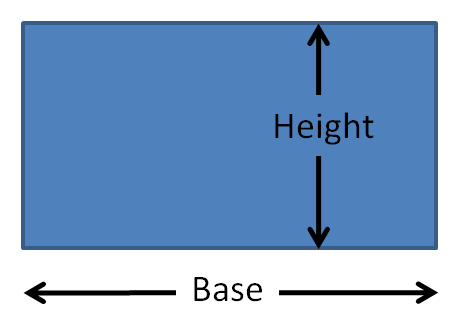You must know:

• the base length
• the perpendicular height from the base to the top.

Area of rectangle = base × height

Now, try this with a piece of paper.Cut off a right-angled triangle from the left-hand side and separate the two pieces.

The area of the two pieces together will be the same as the original rectangle.Move the right-angled triangle over to the right-hand side.

The area still hasn't changed.Join the two pieces to form a parallelogram.

The area of this parallelogram is the same as the original rectangle.Area of ParallelogramsYou must know:

• the base length
• the perpendicular height from the base to the top. (NOT the slant height)

Area of parallelogram = base × height

Example

Calculate the area of this parallelogram in cm2.To calculate th area, we must know:

• the base length
• the perpendicular height from the base to the top. (NOT the slant height)

The base length is 10 cm.

The slant height is 7 cm, which we do not need.

The perpendicular height is 6 cm.

Area = Base × Height = 10 × 6 = 60 cm2

Select the correct formula to calculate the area of a parallelogram.

area = ½ base × height

area = base × perpendicular height

area = base × slant height

Calculate the area of this parallelogram in cm2.

(Just write the number.)Calculate the area of this parallelogram in cm2.

(Just write the number.)Calculate the area of this parallelogram in cm2.

(Just write the number.)Calculate the area of this parallelogram in cm2.

(Just write the number.)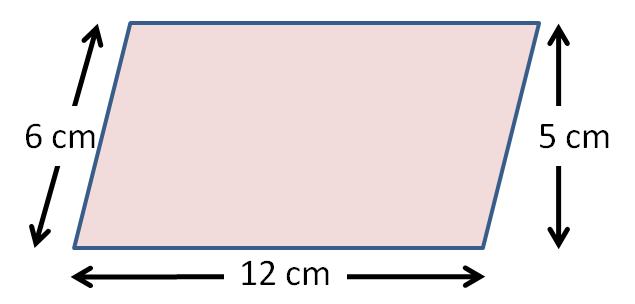Calculate the area of this parallelogram in cm2.

(Just write the number.)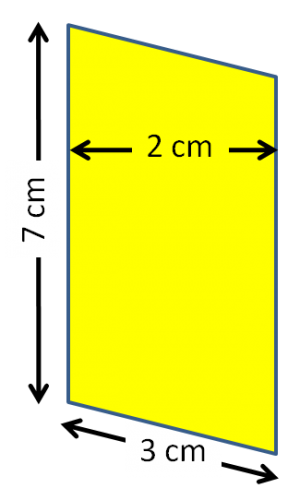Which of these two parallelograms has the larger area?

The base lengths and the perpendicular heights are shown.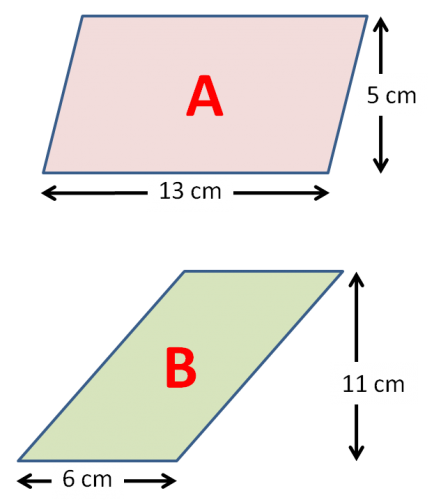A

B

cannot tell

Which of these two parallelograms has the larger area?

The base lengths and the perpendicular heights are shown.A

B

cannot tell

Which of these two parallelograms has the larger area?A

B

cannot tell

Select all the parallelograms which have an area of 160 cm2.A

B

C

• Question 1

Select the correct formula to calculate the area of a parallelogram.

area = base × perpendicular height
EDDIE SAYS
The area uses the perpendicular height, not the slant height.
• Question 2

Calculate the area of this parallelogram in cm2.

(Just write the number.)60
EDDIE SAYS
Base length = 10 cm
Perpendicular height = 6 cm
Area = 10 × 6 = 60 cm2
• Question 3

Calculate the area of this parallelogram in cm2.

(Just write the number.)45
EDDIE SAYS
Base length = 15 cm
Perpendicular height = 3 cm
Area = 15 × 3 = 45 cm2
• Question 4

Calculate the area of this parallelogram in cm2.

(Just write the number.)14
EDDIE SAYS
Base length = 7 cm
Perpendicular height = 2 cm
Area = 7 × 2 = 14 cm2
• Question 5

Calculate the area of this parallelogram in cm2.

(Just write the number.)60
EDDIE SAYS
Base length = 12 cm
Perpendicular height = 5 cm
Area = 12 × 5 = 60 cm2
• Question 6

Calculate the area of this parallelogram in cm2.

(Just write the number.)14
EDDIE SAYS
Base length = 7 cm
Perpendicular height = 2 cm
Area = 7 × 2 = 14 cm2
• Question 7

Which of these two parallelograms has the larger area?

The base lengths and the perpendicular heights are shown.B
EDDIE SAYS
Area of A = 13 × 5 = 65 cm2
Area of B = 6 × 11 = 66 cm2
• Question 8

Which of these two parallelograms has the larger area?

The base lengths and the perpendicular heights are shown.A
EDDIE SAYS
Area of A = 2 × 11 = 22 cm2
Area of B = 7 × 3 = 21 cm2
• Question 9

Which of these two parallelograms has the larger area?cannot tell
EDDIE SAYS
Area of A = 8 × 10 = 80 cm2
We cannot work out the area of B because the perpendicular height is not shown.
• Question 10

Select all the parallelograms which have an area of 160 cm2.A
C
EDDIE SAYS
Area of A = 8 × 20 = 160 cm2
We cannot work out the area of B because the perpendicular height is not shown. Area of C = 16 × 10 = 160 cm2
---- OR ----

Sign up for a £1 trial so you can track and measure your child's progress on this activity.

### What is EdPlace?

We're your National Curriculum aligned online education content provider helping each child succeed in English, maths and science from year 1 to GCSE. With an EdPlace account you’ll be able to track and measure progress, helping each child achieve their best. We build confidence and attainment by personalising each child’s learning at a level that suits them.

Get started